Series Quiz III, Non Verbal Reasoning

# Series Quiz III, Non Verbal Reasoning

Test Description

## 25 Questions MCQ Test General Intelligence and Reasoning for SSC CGL | Series Quiz III, Non Verbal Reasoning

Series Quiz III, Non Verbal Reasoning for SSC CGL 2023 is part of General Intelligence and Reasoning for SSC CGL preparation. The Series Quiz III, Non Verbal Reasoning questions and answers have been prepared according to the SSC CGL exam syllabus.The Series Quiz III, Non Verbal Reasoning MCQs are made for SSC CGL 2023 Exam. Find important definitions, questions, notes, meanings, examples, exercises, MCQs and online tests for Series Quiz III, Non Verbal Reasoning below.
Solutions of Series Quiz III, Non Verbal Reasoning questions in English are available as part of our General Intelligence and Reasoning for SSC CGL for SSC CGL & Series Quiz III, Non Verbal Reasoning solutions in Hindi for General Intelligence and Reasoning for SSC CGL course. Download more important topics, notes, lectures and mock test series for SSC CGL Exam by signing up for free. Attempt Series Quiz III, Non Verbal Reasoning | 25 questions in 50 minutes | Mock test for SSC CGL preparation | Free important questions MCQ to study General Intelligence and Reasoning for SSC CGL for SSC CGL Exam | Download free PDF with solutions
 1 Crore+ students have signed up on EduRev. Have you?
Series Quiz III, Non Verbal Reasoning - Question 1

### Directions to Solve Each of the following questions consists of five figures marked A, B, C, D and E called the Problem Figures followed by five other figures marked 1, 2, 3, 4 and 5 called the Answer Figures. Select a figure from amongst the Answer Figures which will continue the same series as established by the five Problem Figures. Question - Select a figure from amongst the Answer Figures which will continue the same series as established by the five Problem Figures.Problem Figures:                          Answer Figures: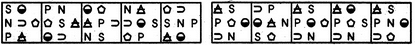(A)     (B)     (C)     (D)     (E)       (1)     (2)     (3)     (4)     (5)

Detailed Solution for Series Quiz III, Non Verbal Reasoning - Question 1 In each step, all the symbols move in the sequence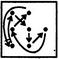Series Quiz III, Non Verbal Reasoning - Question 2

### Directions to Solve Each of the following questions consists of five figures marked A, B, C, D and E called the Problem Figures followed by five other figures marked 1, 2, 3, 4 and 5 called the Answer Figures. Select a figure from amongst the Answer Figures which will continue the same series as established by the five Problem Figures. Question - Select a figure from amongst the Answer Figures which will continue the same series as established by the five Problem Figures.Problem Figures:                          Answer Figures: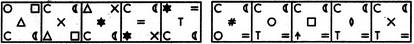(A)     (B)     (C)     (D)     (E)       (1)     (2)     (3)     (4)     (5)

Detailed Solution for Series Quiz III, Non Verbal Reasoning - Question 2 The symbols move in the sequences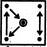and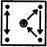alternately. In each step, the symbol that reaches the-encircled position gets replaced by a new symbol.
Series Quiz III, Non Verbal Reasoning - Question 3

### Directions to Solve Each of the following questions consists of five figures marked A, B, C, D and E called the Problem Figures followed by five other figures marked 1, 2, 3, 4 and 5 called the Answer Figures. Select a figure from amongst the Answer Figures which will continue the same series as established by the five Problem Figures. Question - Select a figure from amongst the Answer Figures which will continue the same series as established by the five Problem Figures.Problem Figures:                          Answer Figures: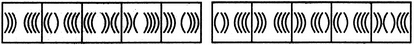(A)     (B)     (C)     (D)     (E)       (1)     (2)     (3)     (4)     (5)

Detailed Solution for Series Quiz III, Non Verbal Reasoning - Question 3 Two, three, four, two, three,..... curves get inverted sequentially.
Series Quiz III, Non Verbal Reasoning - Question 4

Each of the following questions consists of five figures marked A, B, C, D and E called the Problem Figures followed by five other figures marked 1, 2, 3, 4 and 5 called the Answer Figures. Select a figure from amongst the Answer Figures which will continue the same series as established by the five Problem Figures.

Question -

Select a figure from amongst the Answer Figures which will continue the same series as established by the five Problem Figures.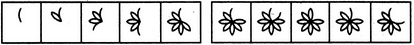(A)     (B)     (C)     (D)     (E)       (1)     (2)     (3)     (4)     (5)
Detailed Solution for Series Quiz III, Non Verbal Reasoning - Question 4 Two and three half-leaves are added to the figure alternately. The addition of half-leaves takes place in an ACW direction.
Series Quiz III, Non Verbal Reasoning - Question 5

Each of the following questions consists of five figures marked A, B, C, D and E called the Problem Figures followed by five other figures marked 1, 2, 3, 4 and 5 called the Answer Figures. Select a figure from amongst the Answer Figures which will continue the same series as established by the five Problem Figures.

Question -

Select a figure from amongst the Answer Figures which will continue the same series as established by the five Problem Figures.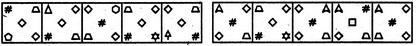(A)     (B)     (C)     (D)     (E)       (1)     (2)     (3)     (4)     (5)
Detailed Solution for Series Quiz III, Non Verbal Reasoning - Question 5 The symbols move in the sequence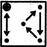in the first step. In each subsequent step, the symbols move in the sequence obtained by rotating the previous sequence through 90oCW. Also, in each step, the symbol that reaches the encircled position gets replaced by a new one.
Series Quiz III, Non Verbal Reasoning - Question 6

Each of the following questions consists of five figures marked A, B, C, D and E called the Problem Figures followed by five other figures marked 1, 2, 3, 4 and 5 called the Answer Figures. Select a figure from amongst the Answer Figures which will continue the same series as established by the five Problem Figures.

Question -

Select a figure from amongst the Answer Figures which will continue the same series as established by the five Problem Figures.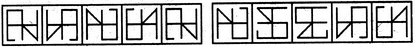(A)     (B)     (C)     (D)     (E)       (1)     (2)     (3)     (4)     (5)
Detailed Solution for Series Quiz III, Non Verbal Reasoning - Question 6 The figure gets laterally inverted and vertically inverted alternately.
Series Quiz III, Non Verbal Reasoning - Question 7

Each of the following questions consists of five figures marked A, B, C, D and E called the Problem Figures followed by five other figures marked 1, 2, 3, 4 and 5 called the Answer Figures. Select a figure from amongst the Answer Figures which will continue the same series as established by the five Problem Figures.

Question -

Select a figure from amongst the Answer Figures which will continue the same series as established by the five Problem Figures.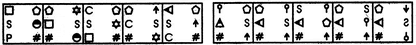(A)     (B)     (C)     (D)     (E)       (1)     (2)     (3)     (4)     (5)
Detailed Solution for Series Quiz III, Non Verbal Reasoning - Question 7 The symbols move in the orders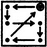and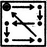alternately. Also, the symbol at the encircled position is replaced by a new one in each step.
Series Quiz III, Non Verbal Reasoning - Question 8

Each of the following questions consists of five figures marked A, B, C, D and E called the Problem Figures followed by five other figures marked 1, 2, 3, 4 and 5 called the Answer Figures. Select a figure from amongst the Answer Figures which will continue the same series as established by the five Problem Figures.

Question -

Select a figure from amongst the Answer Figures which will continue the same series as established by the five Problem Figures.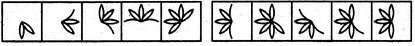(A)     (B)     (C)     (D)     (E)       (1)     (2)     (3)     (4)     (5)
Detailed Solution for Series Quiz III, Non Verbal Reasoning - Question 8 In one step, a half-leaf is added to the figure at the ACW-end. In the next step, two half-leaves are added to the figure - one at the ACW-end and the other at the CW-end. Also, in each step, the figure rotates 45oACW.
Series Quiz III, Non Verbal Reasoning - Question 9

Each of the following questions consists of five figures marked A, B, C, D and E called the Problem Figures followed by five other figures marked 1, 2, 3, 4 and 5 called the Answer Figures. Select a figure from amongst the Answer Figures which will continue the same series as established by the five Problem Figures.

Question -

Select a figure from amongst the Answer Figures which will continue the same series as established by the five Problem Figures.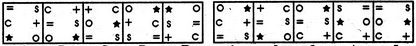(A)     (B)     (C)     (D)     (E)       (1)     (2)     (3)     (4)     (5)
Detailed Solution for Series Quiz III, Non Verbal Reasoning - Question 9 The symbols '=', '+' and '*' move one step ACW While the symbols 's', 'c' and 'o' move one step CW each time.
Series Quiz III, Non Verbal Reasoning - Question 10

Each of the following questions consists of five figures marked A, B, C, D and E called the Problem Figures followed by five other figures marked 1, 2, 3, 4 and 5 called the Answer Figures. Select a figure from amongst the Answer Figures which will continue the same series as established by the five Problem Figures.

Question -

Select a figure from amongst the Answer Figures which will continue the same series as established by the five Problem Figures.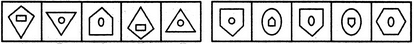(A)     (B)     (C)     (D)     (E)       (1)     (2)     (3)     (4)     (5)
Detailed Solution for Series Quiz III, Non Verbal Reasoning - Question 10 Similar figure repeats in every third step and each time a figure reappears it gets vertically inverted.
Series Quiz III, Non Verbal Reasoning - Question 11

Directions to Solve Each of the following questions consists of five figures marked A, B, C, D and E called the Problem Figures followed by five other figures marked 1, 2, 3, 4 and 5 called the Answer Figures. Select a figure from amongst the Answer Figures which will continue the same series as established by the five Problem Figures.
Question - Select a figure from amongst the Answer Figures which will continue the same series as established by the five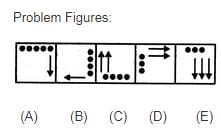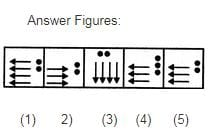Detailed Solution for Series Quiz III, Non Verbal Reasoning - Question 11

In each step, all the existing elements move to the adjacent side (of the square boundary) in a CW direction. The number of black circles decreases by one in first, third, fifth, .... steps and the number of arrows increases by one in second, fourth, sixth,... steps.

Series Quiz III, Non Verbal Reasoning - Question 12

Each of the following questions consists of five figures marked A, B, C, D and E called the Problem Figures followed by five other figures marked 1, 2, 3, 4 and 5 called the Answer Figures. Select a figure from amongst the Answer Figures which will continue the same series as established by the five Problem Figures.

Question -

Select a figure from amongst the Answer Figures which will continue the same series as established by the five Problem Figures.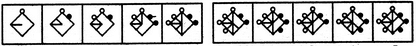(A)     (B)     (C)     (D)     (E)       (1)     (2)     (3)     (4)     (5)
Detailed Solution for Series Quiz III, Non Verbal Reasoning - Question 12 In one step, a black circle is added to the figure at the CW-end of the existing circles and a line segment is added on the upper side. In the next step, a white circle is added to the figure at the ACW-end of the existing circles and a line segment is added on the lower side.
Series Quiz III, Non Verbal Reasoning - Question 13

Each of the following questions consists of five figures marked A, B, C, D and E called the Problem Figures followed by five other figures marked 1, 2, 3, 4 and 5 called the Answer Figures. Select a figure from amongst the Answer Figures which will continue the same series as established by the five Problem Figures.

Question -

Select a figure from amongst the Answer Figures which will continue the same series as established by the five Problem Figures.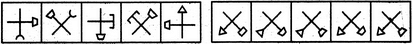(A)     (B)     (C)     (D)     (E)       (1)     (2)     (3)     (4)     (5)
Detailed Solution for Series Quiz III, Non Verbal Reasoning - Question 13 The element having trapezium at its end, rotates 135oACW and the trapezium gets inverted in each step. The other element rotates 135oACW in one step and it rotates 45oCW and the symbol at its end gets replaced by a new element in the next step.
Series Quiz III, Non Verbal Reasoning - Question 14

Each of the following questions consists of five figures marked A, B, C, D and E called the Problem Figures followed by five other figures marked 1, 2, 3, 4 and 5 called the Answer Figures. Select a figure from amongst the Answer Figures which will continue the same series as established by the five Problem Figures.

Question -

Select a figure from amongst the Answer Figures which will continue the same series as established by the five Problem Figures.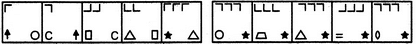(A)     (B)     (C)     (D)     (E)       (1)     (2)     (3)     (4)     (5)
Detailed Solution for Series Quiz III, Non Verbal Reasoning - Question 14 The number of 'L' shaped elements in the upper part of the figure increases by one in every second step. Also, in each step, the existing 'L' shaped elements rotate 90oCW; the lower two elements interchange positions and the element that reaches the lower left corner, gets replaced by a new one.
Series Quiz III, Non Verbal Reasoning - Question 15

Each of the following questions consists of five figures marked A, B, C, D and E called the Problem Figures followed by five other figures marked 1, 2, 3, 4 and 5 called the Answer Figures. Select a figure from amongst the Answer Figures which will continue the same series as established by the five Problem Figures.

Question -

Select a figure from amongst the Answer Figures which will continue the same series as established by the five Problem Figures.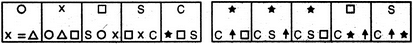(A)     (B)     (C)     (D)     (E)       (1)     (2)     (3)     (4)     (5)
Detailed Solution for Series Quiz III, Non Verbal Reasoning - Question 15 The elements move in the sequences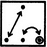and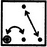alternately. Also, in each step, the element that reaches the encircled position, gets replaced by a new element.
Series Quiz III, Non Verbal Reasoning - Question 16

Each of the following questions consists of five figures marked A, B, C, D and E called the Problem Figures followed by five other figures marked 1, 2, 3, 4 and 5 called the Answer Figures. Select a figure from amongst the Answer Figures which will continue the same series as established by the five Problem Figures.

Question -

Select a figure from amongst the Answer Figures which will continue the same series as established by the five Problem Figures.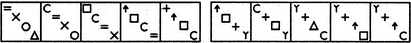(A)     (B)     (C)     (D)     (E)       (1)     (2)     (3)     (4)     (5)
Detailed Solution for Series Quiz III, Non Verbal Reasoning - Question 16 In each step, the symbols move in the sequence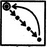and the symbol that reaches the encircled position gets replaced by a new symbol.
Series Quiz III, Non Verbal Reasoning - Question 17

Each of the following questions consists of five figures marked A, B, C, D and E called the Problem Figures followed by five other figures marked 1, 2, 3, 4 and 5 called the Answer Figures. Select a figure from amongst the Answer Figures which will continue the same series as established by the five Problem Figures.

Question -

Select a figure from amongst the Answer Figures which will continue the same series as established by the five Problem Figures.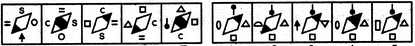(A)     (B)     (C)     (D)     (E)       (1)     (2)     (3)     (4)     (5)
Detailed Solution for Series Quiz III, Non Verbal Reasoning - Question 17 The shading moves upwards sequentially from lower-left corner to the upper-right corner of the rhombus and once it reaches the upper-right corner, it moves to the lower-left corner in the next step. Also, in each step, all the symbols move to the adjacent side (of the rhombus) in a CW direction and the symbol that reaches the LHS position gets replaced by a new symbol.
Series Quiz III, Non Verbal Reasoning - Question 18

Each of the following questions consists of five figures marked A, B, C, D and E called the Problem Figures followed by five other figures marked 1, 2, 3, 4 and 5 called the Answer Figures. Select a figure from amongst the Answer Figures which will continue the same series as established by the five Problem Figures.

Question -

Select a figure from amongst the Answer Figures which will continue the same series as established by the five Problem Figures.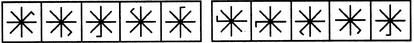(A)     (B)     (C)     (D)     (E)       (1)     (2)     (3)     (4)     (5)
Detailed Solution for Series Quiz III, Non Verbal Reasoning - Question 18 The small line segment attached to the main figure moves 2,1, 3,1, 4,... spaces CW sequentially.
Series Quiz III, Non Verbal Reasoning - Question 19

Each of the following questions consists of five figures marked A, B, C, D and E called the Problem Figures followed by five other figures marked 1, 2, 3, 4 and 5 called the Answer Figures. Select a figure from amongst the Answer Figures which will continue the same series as established by the five Problem Figures.

Question -

Select a figure from amongst the Answer Figures which will continue the same series as established by the five Problem Figures.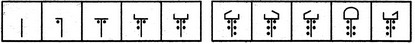(A)     (B)     (C)     (D)     (E)       (1)     (2)     (3)     (4)     (5)
Detailed Solution for Series Quiz III, Non Verbal Reasoning - Question 19 One dot and one line segment are added to the figure in each step, once to the LHS and next to the RHS part of the figure.
Series Quiz III, Non Verbal Reasoning - Question 20

Each of the following questions consists of five figures marked A, B, C, D and E called the Problem Figures followed by five other figures marked 1, 2, 3, 4 and 5 called the Answer Figures. Select a figure from amongst the Answer Figures which will continue the same series as established by the five Problem Figures.

Question -

Select a figure from amongst the Answer Figures which will continue the same series as established by the five Problem Figures.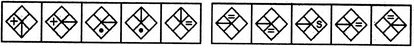(A)     (B)     (C)     (D)     (E)       (1)     (2)     (3)     (4)     (5)
Detailed Solution for Series Quiz III, Non Verbal Reasoning - Question 20 The line segment rotates through 90o and moves to the adjacent portion of the rhombus in an ACW direction in first, third, fifth, ... steps. The other symbol moves to the adjacent portion of the rhombus in an ACW direction and also gets replaced by a new symbol in second, fourth, sixth,... steps.
Series Quiz III, Non Verbal Reasoning - Question 21

Each of the following questions consists of five figures marked A, B, C, D and E called the Problem Figures followed by five other figures marked 1, 2, 3, 4 and 5 called the Answer Figures. Select a figure from amongst the Answer Figures which will continue the same series as established by the five Problem Figures.

Question -

Select a figure from amongst the Answer Figures which will continue the same series as established by the five Problem Figures.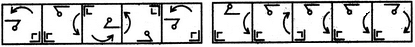(A)     (B)     (C)     (D)     (E)       (1)     (2)     (3)     (4)     (5)
Detailed Solution for Series Quiz III, Non Verbal Reasoning - Question 21 The bent pin gets laterally inverted and vertically inverted alternately and moves to the adjacent side (of the square boundary) in a CW direction in each step,. The curved arrow rotates 90oACW and moves to the adjacent side CW in each step. As for the third element - in one step the outer 'L' shaped symbol gets laterally inverted and in the next step the element gets vertically inverted. Or, similar figure reappears in every fourth step. So, after fig. (E), the fig. (B) should reappear.
Series Quiz III, Non Verbal Reasoning - Question 22

Each of the following questions consists of five figures marked A, B, C, D and E called the Problem Figures followed by five other figures marked 1, 2, 3, 4 and 5 called the Answer Figures. Select a figure from amongst the Answer Figures which will continue the same series as established by the five Problem Figures.

Question -

Select a figure from amongst the Answer Figures which will continue the same series as established by the five Problem Figures.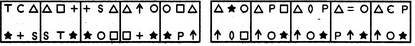(A)     (B)     (C)     (D)     (E)       (1)     (2)     (3)     (4)     (5)
Detailed Solution for Series Quiz III, Non Verbal Reasoning - Question 22 The elements move in the sequences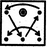and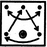alternately. Also, in each step, the element at the encircled position gets replaced by a new element.
Series Quiz III, Non Verbal Reasoning - Question 23

Each of the following questions consists of five figures marked A, B, C, D and E called the Problem Figures followed by five other figures marked 1, 2, 3, 4 and 5 called the Answer Figures. Select a figure from amongst the Answer Figures which will continue the same series as established by the five Problem Figures.

Question -

Select a figure from amongst the Answer Figures which will continue the same series as established by the five Problem Figures.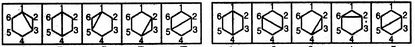(A)     (B)     (C)     (D)     (E)       (1)     (2)     (3)     (4)     (5)
Detailed Solution for Series Quiz III, Non Verbal Reasoning - Question 23 The diagonals of the hexagon are drawn in a sequence - (1, 3), (1, 4), (1, 5), (2, 4), (2, 5), (2, 6), (3, 5), (3, 6), (4, 6).
Series Quiz III, Non Verbal Reasoning - Question 24

Each of the following questions consists of five figures marked A, B, C, D and E called the Problem Figures followed by five other figures marked 1, 2, 3, 4 and 5 called the Answer Figures. Select a figure from amongst the Answer Figures which will continue the same series as established by the five Problem Figures.

Question -

Select a figure from amongst the Answer Figures which will continue the same series as established by the five Problem Figures.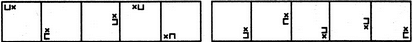(A)     (B)     (C)     (D)     (E)       (1)     (2)     (3)     (4)     (5)
Detailed Solution for Series Quiz III, Non Verbal Reasoning - Question 24 The two elements together move two spaces (each space is equal to half-a-side of the square boundary) and three space ACW alternately. Also, in one step, the two elements interchange positions and together rotate 90oCW and in the next step, the two elements interchange positions and together rotate through 180o.
Series Quiz III, Non Verbal Reasoning - Question 25

Each of the following questions consists of five figures marked A, B, C, D and E called the Problem Figures followed by five other figures marked 1, 2, 3, 4 and 5 called the Answer Figures. Select a figure from amongst the Answer Figures which will continue the same series as established by the five Problem Figures.

Question -

Select a figure from amongst the Answer Figures which will continue the same series as established by the five Problem Figures.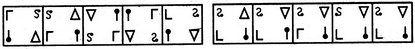(A)     (B)     (C)     (D)     (E)       (1)     (2)     (3)     (4)     (5)
Detailed Solution for Series Quiz III, Non Verbal Reasoning - Question 25 In each step, all the elements move to the adjacent corner (of the square boundary) in an ACW direction. Also, the elements that reach the lower-right and upper-left positions get vertically inverted alternately.

## General Intelligence and Reasoning for SSC CGL

110 videos|66 docs|82 tests
Information about Series Quiz III, Non Verbal Reasoning Page
In this test you can find the Exam questions for Series Quiz III, Non Verbal Reasoning solved & explained in the simplest way possible. Besides giving Questions and answers for Series Quiz III, Non Verbal Reasoning, EduRev gives you an ample number of Online tests for practice

## General Intelligence and Reasoning for SSC CGL

110 videos|66 docs|82 tests(Scan QR code)